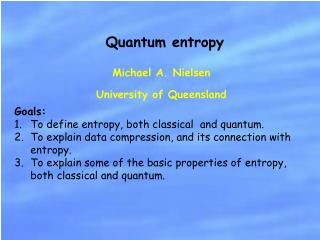DownloadDownload PresentationMichael A. Nielsen University of Queensland

# Michael A. Nielsen University of Queensland

Télécharger la présentation## Michael A. Nielsen University of Queensland

- - - - - - - - - - - - - - - - - - - - - - - - - - - E N D - - - - - - - - - - - - - - - - - - - - - - - - - - -
##### Presentation Transcript

1. Quantum entropy Michael A. Nielsen University of Queensland • Goals: • To define entropy, both classical and quantum. • To explain data compression, and its connection with entropy. • To explain some of the basic properties of entropy, both classical and quantum.

2. What is an information source? 011000101110011100101011100011101001011101000 We need a simple model of an information source. The model might not be realistic, but it should give rise to a theory of information that can be applied to realistic situations.

3. Discrete iid sources 01100010111001110010101110001… Definition: Each output from a discrete information source comes from a finite set. We will mostly be concerned with the case where the alphabet consists of 0 and 1. More generally, there is no loss of generality in supposing that the alphabet is 0,…,n-1.

4. Discrete iid sources 01100010111001110010101110001… We will model sources using a probability distribution for the output of the source. Definition: Each output from an iid (independent and identically distributed) source is independent of the other outputs, and each output has the same distribution. Example: A sequence of coin tosses of a biased coin with probability p of heads, and 1-p of tails. More generally, the distribution on alphabet symbols is denoted p0,p1,…,pn.

5. What other sources are discrete iid? Most interesting sources are not. “What a piece of work is a man! how noble in reason! how infinite in faculties! in form and moving how express and admirable! in action how like an angel! in apprehension how like a god! the beauty of the world, the paragon of animals! And yet to me what is this quintessence of dust?” However, lots of sources can be approximated as iid – even with English text this is not a bad approximation. Many sources can be described as stationary, ergodic sequences of random variables, and similar results apply. Research problem: Find a good quantum analogue of “stationary, ergodic sources” for, and extend quantum information theory to those sources. (Quantum Shannon-Macmillan-Breiman theorem?)

6. How can we quantify the rate at which information is being produced by a source? Two broad approaches Axiomatic approach: Write down desirable axioms which a measure of information “should” obey, and find such a measure. Operational approach: Based on the “fundamental program” of information science. How many bits are needed to store the output of the source, so the output can be reliably recovered?

7. Historical origin of data compression “He can compress the most words into the smallest ideas of any man I ever met.”

8. Data compression abcde… { nR bits abcde… n uses compress decompress What is the minimal value of R that allows reliable decompression? We will define the minimal value to be the information content of the source.

9. Data compression Suppose we flip coins, getting heads with probability p, and tails with probability 1-p. For large values of n, it is very likely that we will get roughly np heads, and n(1-p) tails.

10. Data compression

11. Data compression: the algorithm The two critical facts • x1 • x2 • x3 • x4 • … n+1 bits nH(p,1-p)+1 bits On average, only H(p,1-p) bits were required to store the compressed string, per use of the source.

12. Variants on the data compression algorithm Our algorithm is for large n, gives variable-length output that achieves the Shannon entropy on average. The algorithm never makes an error in recovery. Algorithms for small n can be designed that do almost as well. Fixed-length compression Errors must always occur in a fixed-length scheme, but it does work with probability approaching one.

13. Why it’s impossible to compressbelow the Shannon rate

14. Basic properties of the entropy

15. Why’s this notion called entropy, anyway? From the American Heritage Book of English Usage (1996): “When the American scientist Claude Shannon found that the mathematical formula of Boltzmann defined a useful quantity in information theory, he hesitated to name this newly discovered quantity entropy because of its philosophical baggage. The mathematician John Von [sic] Neumann encouraged Shannon to go ahead with the name entropy, however, since`no one knows what entropy is, so in a debate you will always have the advantage.’ ”

16. What else can be done with the Shannon entropy? • Identify a physical resource – energy, time, bits, space, • entanglement. • Identify an information processing task – data compression, • information transmission, teleportation. 3. Identify a criterion for success. How much of 1 do I need to achieve 2, while satisfying 3? Quantum processes Shor’s algorithm ? teleportation communication quantum phase transitions theory of entanglement cryptography quantum error-correction Complexity

17. What else can be done with the Shannon entropy? Classical processes gambling data compression thermodynamics ? cryptography quantum information networks reliable communication in the presence of noise Complexity

18. What is a quantum information source? Example: “Semiclassical coin toss” Example: “Quantum coin toss”

19. Quantum data compression compression decompression

20. What’s the best possible rate for quantum data compression? “Semiclassical coin toss” “Quantum coin toss”

21. Quantum entropy

22. Basic properties of the von Neumann entropy

23. The typical subspace

24. Outline of Schumacher’s data compression

25. Recall classical to quantum circuits

26. How to measure P, Q

27. Outline of Schumacher’s data compression

28. Outline of Schumacher’s data compression

29. Schumacher compression

30. The idea of the proof is similar to Shannon’s proof. Two known proofs: One is a complicated kludge, done from first principles. The other proof is an elegant “easy” proof that relies on other deep theorems.